# Constructing the Tangents to a Circle from an Exterior Point

Compass and straight edge constructions are of interest to mathematicians, not only in the field of geometry, but also in algebra. For thousands of years, beginning with the Ancient Babylonians, mathematicians were interested in the problem of "squaring the circle" (drawing a square with the same area as a circle) using a straight edge and compass. This problem is equivalent to finding the area of a circle. It turns out that this is impossible, but no-one managed to prove this until 1882!

However, it is possible to construct the tangents to a circle from an exterior point using only a straight edge and compass. For this construction, you will need a straight edge (ruler - but you won't be measuring anything), a pair of compasses, a pencil and paper. I have drawn the pictures using the robocompass app. It's fun to play with, and you can use it to do all sorts of geometric constructions. There's a little bit of coding to learn, but a list of instructions is provided. Once you've written your little program, you can invite a few friends over to watch the construction. Be warned that, when you are using it to draw arcs, the robocompass compass point may appear to be a little away from the centre, but the drawing is actually accurate.

Note: these instructions assume that you are familiar with constructing the perpendicular bisector of a straight line. We need to do that in step 2. If your memories of this construction are a bit hazy, it's probably a good idea to re-read the article on finding the perpendicular bisector of a line segment before trying to do this construction.

## The Construction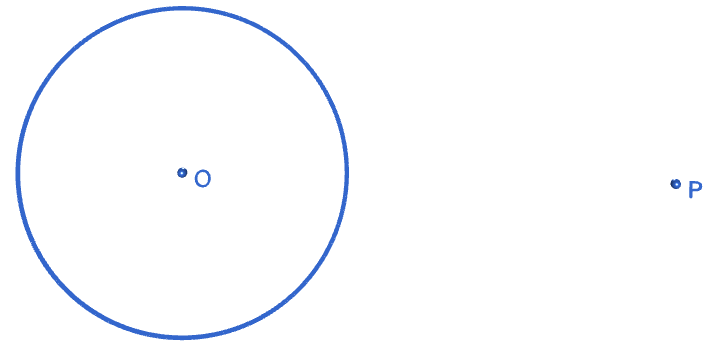Step 1: Start with the circle, with its centre $O$ marked, and the exterior point $P$ that you want your tangents to pass through. If you don't know where the centre of your circle is, don't guess! There's a construction that you can use to find it. Read all about it in the article on constructing the centre of a circle.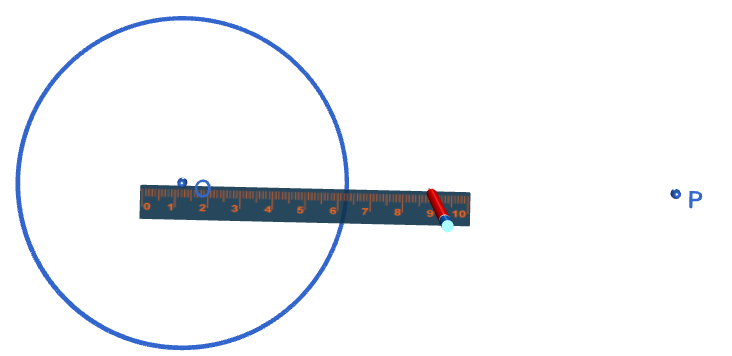Step 2: Use your straight edge to draw a line segment joining the centre $O$ of the circle and the point $P$.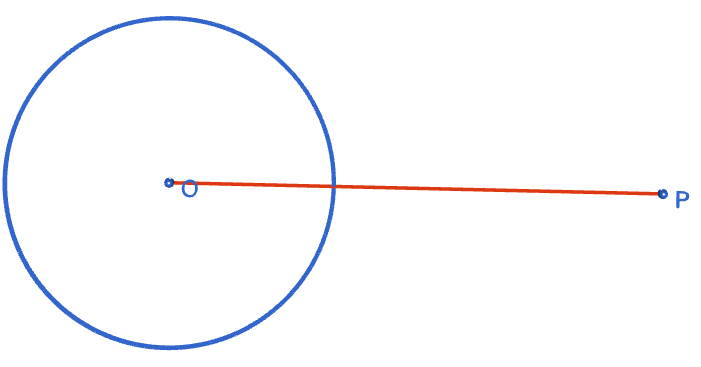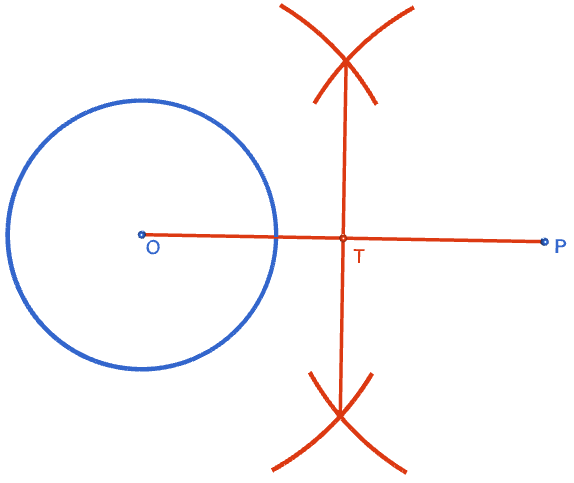Step 3: Construct the perpendicular bisector of line segment $OP$. There's an article on constructing the perpendicular bisector if you've forgotten how to do this. Label the point where the perpendicular bisector crosses the line segment $OP$ with a $T$.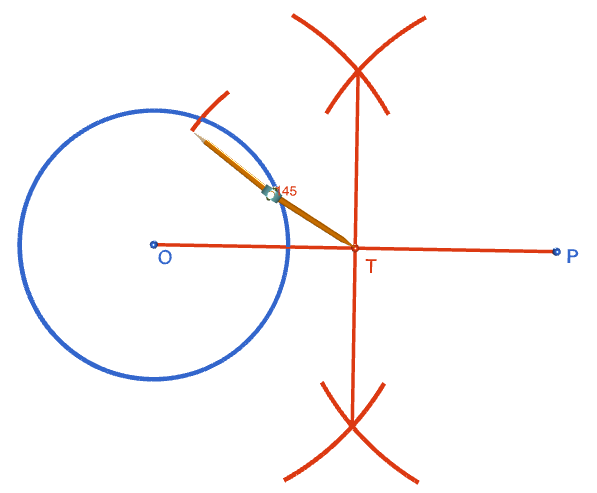Step 4: Open out your compasses to a radius matching the length of line segment $OT$. With the tip of the compasses at point $T$, draw two arcs cutting the circle.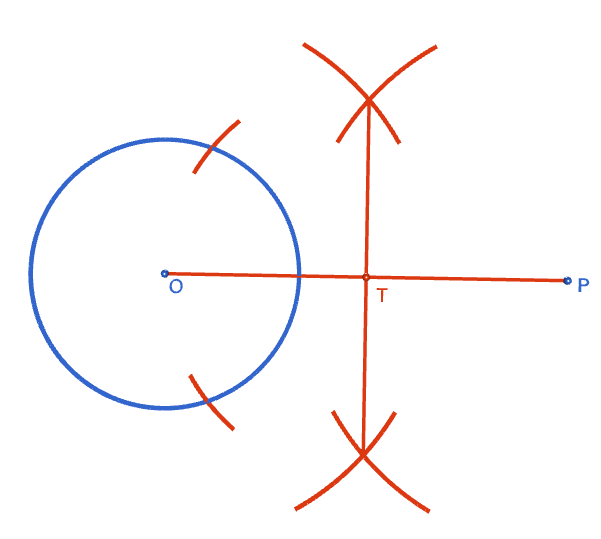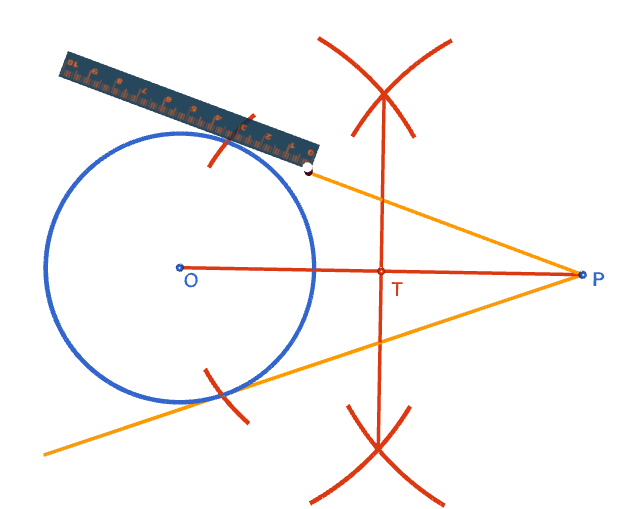Step 5: Using your straight edge, draw two line segments from $P$, one through each point of intersection of the arcs with the circle. These are your tangent lines. The completed construction is shown on the right. Ask your parents to frame your work of art.

What's that, Sam? Your tangents cut the circle at two different places? They aren't tangents then, are they? You might have let your compasses collapse a little between drawing the different arcs.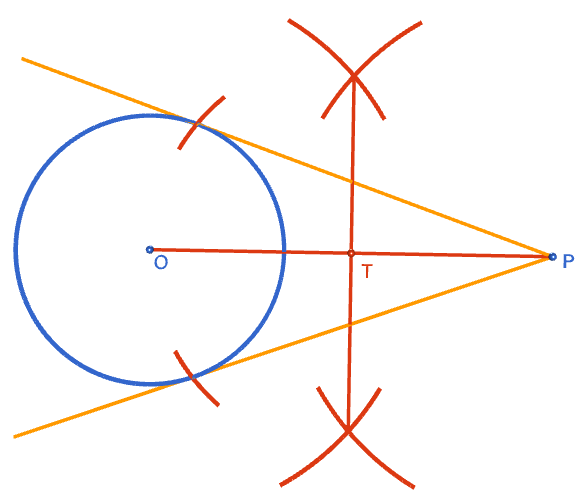### Description

This tutorial will expose you to Rulers and how to use them. We will also have a close look as set square and compass constructions. Your feedback is important to us, if you like any other topic covered under this tutorial, please do let us know.

### Audience

Year 10 or higher

### Learning Objectives

Ruler and compass constructions and more

Author: Subject Coach
You must be logged in as Student to ask a Question.